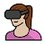# Insertion Sort Questions from Quiz 3

The question is: "In the worst case for a list with 10 distinct elements, how many comparisons are made?"

Why isn't the comparison with the sorted number and the blank left space counted as an individual comparison? I thought that was considered a possible comparison scenario in insertion sorts.

For example, sorting 3, 2, 1:

• 1st round: 2 < 3, (blank) 2 = 2 comparisons
• 2nd round: 1 < 3, 1 < 2, (blank) 1 = 3 comparisons

For a total of 5 comparisons.

Right?Note by Anna Morales
3 years, 2 months ago

This discussion board is a place to discuss our Daily Challenges and the math and science related to those challenges. Explanations are more than just a solution — they should explain the steps and thinking strategies that you used to obtain the solution. Comments should further the discussion of math and science.

When posting on Brilliant:

• Use the emojis to react to an explanation, whether you're congratulating a job well done , or just really confused .
• Ask specific questions about the challenge or the steps in somebody's explanation. Well-posed questions can add a lot to the discussion, but posting "I don't understand!" doesn't help anyone.
• Try to contribute something new to the discussion, whether it is an extension, generalization or other idea related to the challenge.

MarkdownAppears as
*italics* or _italics_ italics
**bold** or __bold__ bold
- bulleted- list
• bulleted
• list
1. numbered2. list
1. numbered
2. list
Note: you must add a full line of space before and after lists for them to show up correctly
paragraph 1paragraph 2

paragraph 1

paragraph 2

[example link](https://brilliant.org)example link
> This is a quote
This is a quote
    # I indented these lines
# 4 spaces, and now they show
# up as a code block.

print "hello world"
# I indented these lines
# 4 spaces, and now they show
# up as a code block.

print "hello world"
MathAppears as
Remember to wrap math in $$ ... $$ or $ ... $ to ensure proper formatting.
2 \times 3 $2 \times 3$
2^{34} $2^{34}$
a_{i-1} $a_{i-1}$
\frac{2}{3} $\frac{2}{3}$
\sqrt{2} $\sqrt{2}$
\sum_{i=1}^3 $\sum_{i=1}^3$
\sin \theta $\sin \theta$
\boxed{123} $\boxed{123}$

Sort by:

The only way I can justify this is that blank comparisons are not considered individual comparisons because they do not cause the cycle to repeat. In mind, the computer would perform the blank comparison and just be like "oh, nothing to compare, I'm all good" and thus this minor comparison was just to check for another number. So since, there were no numbers to compare, it is not a full comparison.

Does this make sense? I'm trying to justify the $\frac{(n-1)(n)}{2}$ formula. (Also, if anyone can link the theory to that formula, that would be great!)

- 3 years, 2 months ago

The formula is sum of first $n-1$ natural numbers. It is also the number of ways to choose 2 things from a set of $n$ things, see combinations

- 3 years, 2 months ago

Thanks!

- 3 years, 2 months ago

Can you explain what is this blank comparison that you are referring to?

- 3 years, 2 months ago

Sure! So when Insertion Sort was introduced, this was what I was told:

Insertion Sort method will place a single element x into a sorted array A. First, x is placed at the end of A. Second, x is compared to the element on the left, there are 3 possible scenarios:

1. There is no element to the left, so the process is finished because x is the smallest element and is already at the start of the array

2. x is greater than or equal to the left element, so the process is finished

3. x is less than the left element = positions are switched

Thirdly, the cycle repeats until x meets scenario 1 or 2.

The blank comparison I'm referring to is scenario 1, when there is no left element.

- 3 years, 2 months ago

You are indeed correct. Insertion sort involves repeating the procedure above for every element of the input array.

Now, it is possible that you might get a slightly different number of comparisons based on what you define to be comparisons. However, we are interested in getting a rough approximation of how this number depends on the size of input. So, usually just noticing that the number of comparisons is $O(n^2)$ is good enough.

If that answer confuses you further, let me know.

- 3 years, 2 months ago